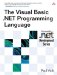# Subroutines and Functions

 <  Day Day Up  >

 A method is either a function or a subroutine . A subroutine performs some action and returns. For example: ` Sub Main() Console.WriteLine("Hello, world!") End Sub ` A function , on the other hand, calculates a value that is returned to the caller when the function ends. When a function is declared, it specifies the type of the value that it returns in much the same way that a variable specifies its type. For example, the following function calculates the sum of two numbers and returns the result. ` Function Add(ByVal x As Integer, ByVal y As Integer) As Integer Return x + y End Function ` If the type of a function is omitted, the type is assumed to be Object . The Option Strict statement at the beginning of a source file requires that all variable declarations have an explicit type. Values can be returned from functions in one of two ways. One way is by using the Return statement, as discussed in Chapter 6, Statements. Alternatively, every function implicitly declares a local variable with the same name as the function itself to hold the return value of the function. This variable is called the function return variable . The previous example could have been written as follows . ` Function Add(ByVal x As Integer, ByVal y As Integer) As Integer Add = x + y End Function ` When the function exits, the value stored in the Add function return variable is returned as the value of the function.
 <  Day Day Up  >The Visual Basic .NET Programming Language
ISBN: 0321169514
EAN: 2147483647
Year: 2004
Pages: 173
Authors: Paul Vick

Similar book on Amazon